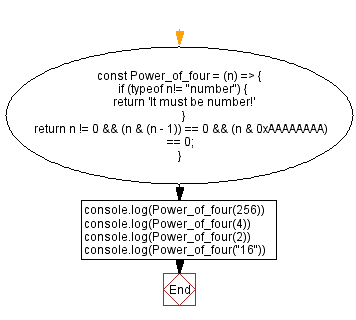# JavaScript - Check a number is a power of 4 or not

## JavaScript Bit Manipulation: Exercise-6 with Solution

Write a JavaScript program to check if a given positive number is a power of four or not using bit manipulation.

The expression n & (n-1) will unset the rightmost set bit of a number. If the number is a power of 2, it has only a 1-bit set, and n & (n-1) will unset the only set bit. So, we can say that n & (n-1) returns 0 if n is a power of 2; otherwise, it's not a power of 2.
The mask 0xAAAAAAAA will allow us to determine where the set bit is located. In all of its odd positions, the mask 0xAAAAAAAA has a value of 1. The set bit in n will be even if the expression (n & 0xAAAAAAAA) is true.
(0xAAAAAAAA)16 = (10101010101010101010101010101010)2.

Test Data:
(256) -> true
(4) -> true
(2) -> false
("16") -> "It must be number!"

Sample Solution:

JavaScript Code:

``````const Power_of_four = (n) => {
if (typeof n!= "number") {
return 'It must be number!'
}
return n != 0 && (n & (n - 1)) == 0 && (n & 0xAAAAAAAA) == 0;
}
console.log(Power_of_four(256))
console.log(Power_of_four(4))
console.log(Power_of_four(2))
console.log(Power_of_four("16"))
```
```

Sample Output:

```true
true
false
It must be number!
```

Flowchart:Live Demo:

See the Pen javascript-bit-manipulation-exercise-6 by w3resource (@w3resource) on CodePen.

* To run the code mouse over on Result panel and click on 'RERUN' button.*

Improve this sample solution and post your code through Disqus

What is the difficulty level of this exercise?

Test your Programming skills with w3resource's quiz.

﻿